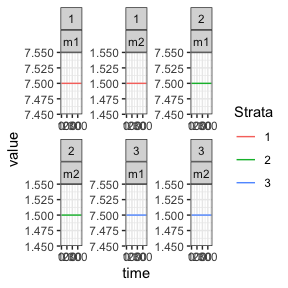# Hybrid MoI (Multiplicity of Infection) Human Model

This is a hybrid model which tracks the mean multiplicity of infection (superinfection) in two compartments. The first, $$m1$$ is all infections, and the second $$m2$$ are apparent (patent) infections. Therefore $$m2$$ is “nested” within $$m1$$. It is a “hybrid” model in the sense of Nåsell (1985).

# Differential Equations

The equations are as follows:

$\dot{m_{1}} = h - r_{1}m_{1}$ $\dot{m_{2}} = h - r_{2}m_{2}$ Where $$h = b EIR$$, is the force of infection. Prevalence can be calculated from these MoI values by:

$x_{1} = 1-e^{-m_{1}}$ $x_{2} = 1-e^{-m_{2}}$ The net infectious probability to mosquitoes is therefore given by:

$x = c_{2}x_{2} + c_{1}(x_{1}-x_{2})$

Where $$c_{1}$$ is the infectiousness of inapparent infections, and $$c_{2}$$ is the infectiousness of patent infections.

# Equilibrium solutions

One way to proceed is assume that $$m_{2}$$ is known, as it models the MoI of patent (observable) infections. Then we have:

$h = r_{2}/m_{2}$ $m_{1} = h/r_{1}$ We can use this to calculate the net infectious probability, and then $$\kappa = x \cdot H$$, allowing the equilibrium solutions of this model to feed into the other components.

# Example

library(exDE)
library(deSolve)
library(data.table)
library(ggplot2)

Here we run a simple example with 3 population strata at equilibrium. We use exDE::make_parameters_X_hMoI to set up parameters. Please note that this only runs the human population component and that most users should read our fully worked example to run a full simulation.

nStrata <- 3
H <- c(100, 500, 250)
b <- 0.55
c1 <- 0.05
c2 <- 0.25
r1 <- 1/250
r2 <- 1/50
Psi <- matrix(data = 1,nrow = 1, ncol = nStrata)

m20 <- 1.5
h <- r2*m20
m10 <- h/r1

EIR <- h/b

params <- list(
nStrata = nStrata
)
params <- list2env(params)

make_parameters_X_hMoI(pars = params, b = b, c1 = c1, c2 = c2, r1 = r1, r2 = r2, Psi = Psi, m10 = m10, m20 = m20, H = H)
make_indices(params)

y0 <- rep(0, 6)
y0[params$m1_ix] <- m10 y0[params$m2_ix] <- m20

out <- deSolve::ode(y = y0, times = c(0, 365), func = function(t, y, pars, EIR) {
list(dXdt(t, y, pars, EIR))
}, parms = params, method = 'lsoda', EIR = as.vector(EIR))

colnames(out)[params$m1_ix+1] <- paste0('m1_', 1:params$nStrata)
colnames(out)[params$m2_ix+1] <- paste0('m2_', 1:params$nStrata)

out <- as.data.table(out)
out <- melt(out, id.vars = 'time')
out[, c("Component", "Strata") := tstrsplit(variable, '_', fixed = TRUE)]
out[, variable := NULL]

ggplot(data = out, mapping = aes(x = time, y = value, color = Strata)) +
geom_line() +
facet_wrap(Strata ~ Component, scales = 'free') +
theme_bw()